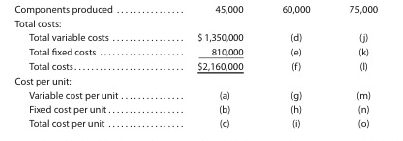Chapter 21, Problem 21.6EX

Chapter
Section
Textbook Problem

Relevant range and fixed and variable costsVogel Inc. manufactures memory chips for electronic toys within a relevant range of 45,000 to 75,000 memory chips per year. Within this range, the following partially completedManufacturing cost schedule has been preparedComplete the cost schedule, identifying each cost by the appropriate letter (a) through (o).

To determine

Variable costs: These are the costs that proportionately change with the changes in the activity base such as units of production Common examples of variable costs are direct materials and direct labor costs.

Fixed Costs: These are the costs that remain constant in total dollar amount irrespective to the changes in the activity base such as units of production. Common examples of fixed costs are factory overhead costs and straight-line depreciation expenses.

To complete: the cost schedule by identifying each cost by the appropriate letter (a) through (o).

Explanation

Complete the cost schedule by identifying each cost by the appropriate letter (a) through (o).

 Cost Schedule Components produced (units) 45,000 60,000 75,000 Total costs: Total variable costs $1,350,000 (d)$1,800,000 (j) $2,250,000 Total fixed costs$810,000 (e)     $810,000 (k)$810,000 Total costs $2,160,000 (f)$2,160,000 (l)  $3,060,000 Cost per unit: Variable cost per unit (a)$30.00 (g)         $30.00 (m)$30.00 Fixed cost per unit (b)    $18.00 (h)$13.50 (n)         $10.80 Total cost per unit (c)$48.00 (i)          $43.50 (o)$40.80

Table (1)

Working notes:

(a)

Determine the variable cost per unit for 45,000 units.

Total variable cost =$1,350,000 Number of units =45,000 Variablecostperunit =TotalvariablecostNumberofunitsproduced=$1,350,00045,000units=$30.00perunit (b) Determine the fixed cost per unit for 45,000 units. Total fixed cost =$810,000

Numver of units =45,000

Fixedcostperunit =TotalfixedcostNumberofunitsproduced=$810,00045,000units=$18.00perunit

(c)

Determine the total cost per unit.

Variable cost per unit =$30.00 per unit (refer (a)) Fixed cost per unit =$18.00 per unit (refer (b))

Totalcostperunit =(Variablecostperunit)+(Fixedcostperunit)=$30.00+$18.00=$48.00perunit (d) Determine the total variable cost for 60,000 units. Variable cost per unit =$30.00 per unit (refer (a))

Numver of units =60,000

Totalvariablecost =(Variablecostperunit)×(Numberofunitsproduced)=$30.00perunit×60,000units=$1,800,000

(e)

Determine the total fixed cost for 60,000 units.

Total fixed cost always remains same irrespective to the number of units produced. As given, the fixed cost for 45,000 units is $810,000. Thus for 60,000 units, the amount of fixed cost would remain same, that is$810,000.

(f)

Determine the total costs.

Total variable cost for 60,000 units =$1,800,000 (refer (d)) Total fixed cost for 60,000 =$810,000 (refer (e))

Totalcost =(TotalVariablecost)+(TotalFixedcost)=$1,800,000+$810,000=$2,610,000 (g) Determine the variable cost per unit for 60,000 units. Variable cost per unit do not change with the change in the number of units produced. Total variable cost =$1,800,000

Numver of units =60,000

Variablecostperunit =TotalvariablecostNumberofunitsproduced=$1,800,00060,000units=$30

Still sussing out bartleby?

Check out a sample textbook solution.

See a sample solution

The Solution to Your Study Problems

Bartleby provides explanations to thousands of textbook problems written by our experts, many with advanced degrees!

Get Started